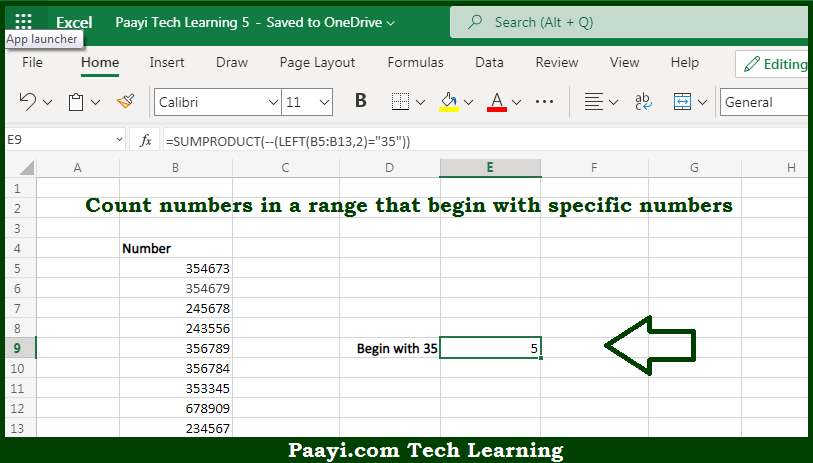# Learn How to Count Number That Begin With Specific Number in Microsoft Excel

Written by | 0 Comments | 466 Views

In this article, you will learn how to COUNT various things in Microsoft Excel using a single or combination of functions and its purpose in Microsoft Excel. You will also get to know how to Count Number That Begins With a Specific Number

Count Number That Begin With Specific Number in Microsoft Excel

The main purpose of this formula is to count numbers in a range that begins with specific numbers. Here we will learn how to count numbers that begin with a certain number in Microsoft Excel. That implies, with the help of a formula based on the SUMPRODUCT and LEFT functions you can able to count numbers in a range that begins with specific numbers. So, with the help of this formula, you can able to count numbers that begin with a certain number.

General Formula to Count Number That Begin With Specific Number

=SUMPRODUCT(--(LEFT(range,chars)="xx"))

The Explanation for the Count Number That Begin With Specific NumberSo we know that with the help of the given formula above you can able to count numbers in a range that begins with specific numbers. Here we will learn how to count numbers that begin with a certain number in Microsoft Excel. As we know that, the SUMPRODUCT  function is very versatile and efficient and works very well with the arrays. Here we are using the LEFT function inside the SUMPRODUCT function as shown in the above formula. So, with the help of this formula, you can able to count numbers in a range that begins with specific numbers.